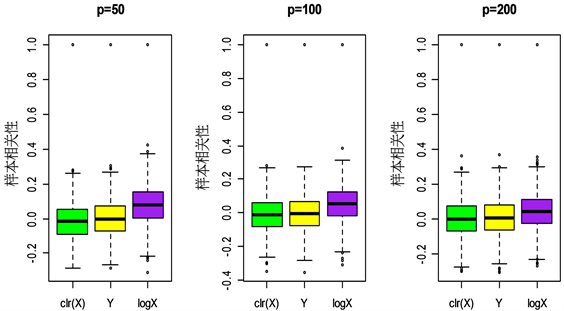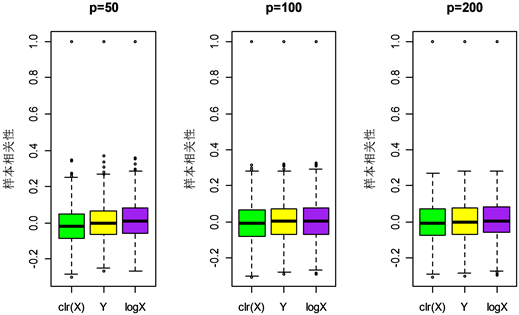# 高维成分数据的精度矩阵估计Large Precision Matrix Estimation for Compositional Data

DOI: 10.12677/SA.2019.85088, PDF, HTML, XML, 下载: 424  浏览: 713  国家科技经费支持

Abstract: High-dimensional compositional data arise in many applications, and statistical methods often fail to produce sensible results due to the unit-sum constraints. The estimation of high dimensional covariance matrix or precision (inverse covariance) matrix is the basic problem of modern multivariate analysis. In this paper, the precision matrix estimation problem for high-dimensional compositional data is considered. It is known that the inverse of the sample covariance matrix is unstable for the estimate precision matrix. Since the sample size of the data is smaller than the number of variables, the inverse of the high-dimensional data matrix is difficult to estimate. In this paper, we use the centered log-ratio transformation method to process high-dimensional compositional data, and then solve the singularity problem of covariance matrix, and obtain the precision matrix estimation of high-dimensional compositional data. Simulation experiments and actual data can verify the rationality of the proposed method.

1. 引言

2. 方法

2.1. 记号

2.2. 方法

${S}^{p-1}=\left\{X={\left({X}_{1},{X}_{2},\cdots ,{X}_{p}\right)}^{\text{T}};{X}_{i}>0,i=1,2,\cdots ,p;\underset{i=1}{\overset{p}{\sum }}{X}_{i}=1\right\}$，其中 $X={\left({X}_{1},\cdot \cdot \cdot ,{X}_{p}\right)}^{\text{T}}$ 是p维成分数据， ${S}^{p-1}$$p-1$ 维单形空间。对成分数据做对数比变换，把成分单形空间映射到欧几里得空间中，从而使经典的统计方法可以适用于变换后的数据。在此我们采用中心对数比变换：

$clr\left(X\right)=\left(\mathrm{log}\frac{{X}_{1}}{g\left(X\right)},\cdots ,\mathrm{log}\frac{{X}_{p}}{g\left(X\right)}\right)$ (1)

$g\left(X\right)={\left(\underset{i=1}{\overset{p}{\prod }}{X}_{i}\right)}^{1/p}$ 是X的几何均值。

${S}_{i}=clr\left(Xi\right)$

${\gamma }_{jk}=\mathrm{cov}\left({S}_{j},{S}_{k}\right)$ (2)

${f}_{i}\left(\stackrel{^}{\Gamma },B\right)=\frac{1}{2}{\beta }_{i}^{\text{T}}\stackrel{^}{\Gamma }{\beta }_{i}-{\beta }_{i}^{\text{T}}{e}_{i}$ (3)

$\stackrel{^}{\Gamma }$ 是正定的，则 ${f}_{i}$ 是凸函数，当损失函数趋于0时，损失函数越小，B越趋近 $\Omega$

$\frac{1}{2}{\beta }^{\text{T}}\stackrel{^}{\Gamma }\beta -{e}_{i}^{\text{T}}\beta +{\lambda }_{ni}{|\beta |}_{1}$ (4)

${\stackrel{^}{\beta }}_{i}$ 是下式的解

${\stackrel{^}{\beta }}_{i}=\underset{\beta \in {R}^{p}}{\mathrm{arg}\mathrm{min}}\left\{\frac{1}{2}{\beta }^{\text{T}}\stackrel{^}{\Gamma }\beta -{e}_{i}^{\text{T}}\beta +{\lambda }_{ni}{|\beta |}_{1}\right\}$ (5)

$\stackrel{^}{B}=\left({\stackrel{^}{\beta }}_{1},\cdot \cdot \cdot ,{\stackrel{^}{\beta }}_{p}\right)$，其中 ${\stackrel{^}{\beta }}_{i}={\left({\stackrel{^}{\beta }}_{i1},\cdot \cdot \cdot ,{\stackrel{^}{\beta }}_{ip}\right)}^{\text{T}}$

${\stackrel{^}{\lambda }}_{i}=\underset{0\le j\le N}{\mathrm{arg}\mathrm{min}}\left\{\frac{1}{H}\underset{v=1}{\overset{H}{\sum }}\left[\frac{1}{2}{\left({\stackrel{^}{\beta }}_{i}^{-v}\left({\lambda }_{j}\right)\right)}^{\text{T}}{\stackrel{^}{\Gamma }}^{v}{\stackrel{^}{\beta }}_{i}^{-v}\left({\lambda }_{j}\right)-{e}_{i}^{\text{T}}{\stackrel{^}{\beta }}_{i}^{-v}\left({\lambda }_{j}\right)\right]\right\}$ (6)

$\stackrel{^}{\Omega }={\left({\stackrel{^}{\omega }}_{ij}\right)}_{p×p}$ 其中 ${\stackrel{^}{\omega }}_{ij}={\stackrel{^}{\omega }}_{ji}={\stackrel{^}{\beta }}_{ij}I\left\{|{\stackrel{^}{\beta }}_{ij}|<|{\stackrel{^}{\beta }}_{ji}|\right\}+{\stackrel{^}{\beta }}_{ji}I\left\{|{\stackrel{^}{\beta }}_{ij}|\ge |{\stackrel{^}{\beta }}_{ji}|\right\}$

3. 数值模拟

${W}_{kj}={\text{e}}^{{Y}_{kj}}$${X}_{kj}={W}_{kj}/\underset{i=1}{\overset{p}{\sum }}{W}_{ki},j=1,\cdots ,p$ (7)Figure 1. Boxplots of sample correlation under different transformations in mode 1Figure 2. Boxplots of sample correlation under different transformations in mode 2Table 1. The precision matrix performance index under different transformations obtained in mode 1Table 2. The precision matrix performance index under different transformations obtained in mode 2

4. 与炎症性肠病(IBD)相关的细菌物种数据集分析

IBD数据集收集了85例IBD病例的粪便样本和26个正常对照样本，并对每个样品进行宏基因组测序，从而鉴定出总共97种细菌物种  。对于数据集中的零元素，在不超过数据生成过程中的最小探测精度的条件下，我们取103。取正常样本( $k=1$ )的1/5，病例样本( $k=2$ )的1/5组成测试集，其他样本组成训练集。然后我们对数据集进行线性判别分析，其分析模型可以参见文献  。

${\delta }_{k}\left(X\right)={X}^{\text{T}}\stackrel{^}{\Omega }{\stackrel{^}{\mu }}_{k}-\frac{1}{2}{\stackrel{^}{\mu }}_{k}^{\text{T}}\stackrel{^}{\Omega }{\stackrel{^}{\mu }}_{k}+\mathrm{log}{\stackrel{^}{\pi }}_{k}$ (8)

$TPR=\frac{TP}{TP+FN}$$FPR=\frac{FP}{FP+TN}$ (9)

$MCC=\frac{TP×TN-FP×FN}{\sqrt{\left(TP+FP\right)\left(TP+FN\right)\left(TN+FP\right)\left(TN+FN\right)}}$ (10)Table 3. Classification performance result

5. 结语

  Ferrers, N.M. (1866) An Elementary Treatise on Trilinear Coordinates. Macmillan, London.  Aitchison, J. (1968) The Statistical Analysis of Compositional Data. Chapman and Hall, London.  Aitchison, J. (1994) A Concise Guide to Compositional Data Analysis. Institute of Mathematical Statistics Lecture Notes—Monograph Series, Vol. 24, 73-81. https://doi.org/10.1214/lnms/1215463786  Aitchison, J. and Egozcue, J.J. (2005) Compositional Data Analysis: Where Are We and Where Should We Be Heading. Mathematical Geology, 37, 829-850. https://doi.org/10.1007/s11004-005-7383-7  Egozcue, J.J., Pawlowsky-Glahn, V., Mateu-Figueras, G., et al. (2003) Isometric Logratio Transformations for Compositional Data Analysis. Mathematical Geology, 35, 279-300. https://doi.org/10.1023/A:1023818214614  Wang, H., Liu, Q., Henry, M.K., et al. (2007) A Hyperspherical Transformation Forecasting Model for Compositional Data. European Journal of Operational Research, 179, 459-468. https://doi.org/10.1016/j.ejor.2006.03.039  Bickel, P.J. and Levina, E. (2008) Covariance Regularization by Thresholding. Annals of Statistics, 36, 2577-2604. https://doi.org/10.1214/08-AOS600  Rothman, A.J., Levina, E. and Zhu, J. (2009) Generalized Thresholding of Large Covariance Matrices. Journal of the American Statistical Association, 104, 177-186. https://doi.org/10.1198/jasa.2009.0101  Cai, T. and Liu, W. (2011) Adaptive Thresholding for Sparse Covariance Matrix Estimation. Journal of the American Statistical Association, 106, 672-684. https://doi.org/10.1198/jasa.2011.tm10560  Friedman, J., Hastie, T. and Tibshirani, R. (2008) Sparse Inverse Covariance Estimation with the Graphical Lasso. Biostatistics, 9, 432-441. https://doi.org/10.1093/biostatistics/kxm045  Cai, T., Liu, W. and Luo, X. (2011) A Constrained L1 Minimization Approach to Sparse Precision Matrix Estimation. Journal of the American Statistical Association, 106, 594-607. https://doi.org/10.1198/jasa.2011.tm10155  Liu, W. and Luo, X. (2015) Fast and Adaptive Sparse Precision Matrix Estimation in High Dimensions. Journal of Multivariate Analysis, 135, 153-162. https://doi.org/10.1016/j.jmva.2014.11.005  Fan, J., Liao, Y. and Liu, H. (2016) An Overview of the Estimation of Large Covariance and Precision Matrices. The Econometrics Journal, 19, C1-C32. https://doi.org/10.1111/ectj.12061  Cao, Y., Lin, W. and Li, H. (2018) Large Covariance Estimation for Compositional Data via Composition-Adjusted Thresholding. Journal of the American Statistical Association, 114, 759-772.  Lu, J.R., Shi, P.X. and Li, H.Z. (2018) Generalized Linear Models with Linear Constraints for Microbiome Compositional Data. Biometrics.